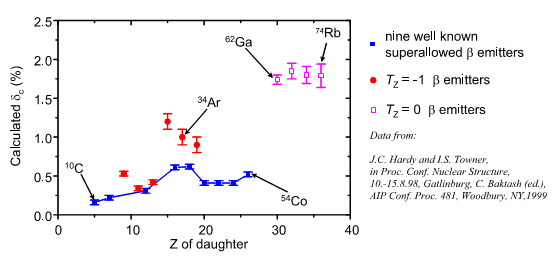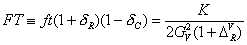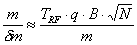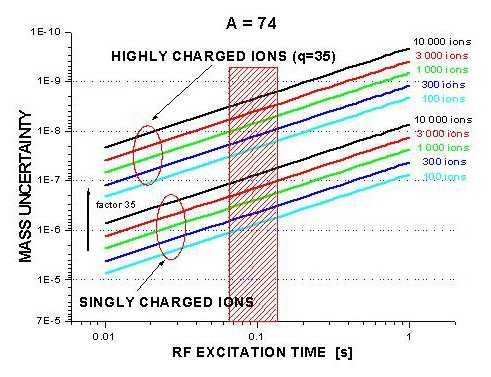High Accuracy Mass Measurements for Fundamental Symmetry Studies

The binding energy of the atomic nucleus is certainly one of the most fundamental properties of such many body systems. Accurate mass measurements serve as a testing ground for nuclear models and stimulate their further improvement, and are required for weak interaction studies in nuclear β-decay. Accurate mass measurements of high precision (δm /m < 10-8) on very short-lived isotopes (T1/2 50 ms) are needed to experimentally test the theoretical corrections, which are employed to determine Vud, the dominant matrix element of the Cabibbo-Kobayashi-Maskawa (CKM) quark mixing matrix. The CKM matrix should be unitary, based on fundamental concepts, however, the present experimental results lead to Vud2+ Vus2+ Vub2 = 0.9740 ± 0.0014,  which points to a 2.2 sigma deviation from the expected value. High accuracy mass measurements would help to test the applied theoretical corrections for the determination of Vud, and may ultimately help determine, if the deviation from unitarity is due to the reached precision or a nature-made effect. Should the effect be intrinsic to nature, this would strongly imply physics beyond the Standard Model.Fig 2: Calculated contribution of theoretical corrections dC to the ft value. The data of the nine well known superallowed b emitters are connected, where the others, not connected points are the new proposed b emitters, where complete experimental data sets not yet exist.The experimental data needed are the ft values for superallowed b transitions. These allow one to determine Gv, the vector coupling constant, which then can be used to determine the Vud matrix element for the up-down quark mixing. Gv can basically be obtained from the experimental ft values and employing the well-known relationship which is true for 0+ ® 0+  b transitions.  f is the statistical rate function, t is the partial half-life for the transition. FT is defined as the corrected ft value, were isospin symmetry breaking correction dC and the transition-dependent part of radiative correction dR are applied. DVR  is the radiative correction part that is independent of the transition, and K is a constant. In order to be able to determine the source of the deviation from unitary, one needs to exclude the possibility of erroneous theoretical corrections. To test this, the experimental precision has to be better than the theoretical corrections applied . For the partial half-live, the experimental achievements have been demonstrated on this level of precision  and are even further developed .

The experimental requirements for the statistical rate function f can be determined in the following way: fµ Q5, and δf/f µ 5δQ/Q, where Q is defined as the total available energy for the nuclear decay, hence the mass difference between the mother and daughter nucleus, minus the electron rest mass. In order to test the theoretical corrections on the necessary level needed, is δf/f < 0.1% that means δQ/Q < 0.02%, or in absolute values, to give an example for the case of 74Rb, where Q = 10.4 MeV, δQ < 2 keV, corresponding to a mass uncertainty for mother and daughter nucleus of δm /m < 210-8. The case of 74Rb would clearly be the first mass measurement for TITAN, since the other needed properties of this nucleus were already determined at ISAC. Other candidates for TITAN measurements are listed in table 1, together with predicted ISAC yields. In the first series of these measurements one would focus on the new list of odd-Z, Tz=0  b emitters, with A ³ 62. The motivation for precision measurements in these nuclei comes from the fact that the calculated values  of the corrections are larger than those for the nine well know transitions nuclei.

If the experimental precision can be reached this would clearly be a valuable test of the accuracy of the theoretical corrections, specifically of the dC part. The second series of measurements would focus on the enhancement in precision in the already fairly well known masses of the nuclei with even-Z, Tz=-1 b emitters, with 18 <A <42. The corrections in this series of transitions are smaller than for the heavier ones, but still larger than for the nine well known candidates. By reaching the better experimental precision, the results from these measurements will help understand the underlying concepts of the corrections.

Table 1. List of envisaged candidates for precision mass measurements at TITAN. The # indicates, that the mass is derived from extrapolations of systematic trends. The expected yield and year is given, together with the production target and ion-source (LIS=Laser Ion Source).

 Isotope Half-live Present δm Expected yield (year) Production odd-Z, Tz=0 b emitters, with A ³ 62 62Ga 116 ms 28 keV 5103                         2004 Zr    LIS 62Zn 9.1 h 10 keV 5103                         2004 Zr    LIS 66As 96 ms 200 keV 1104                         2003 Zr    ECR 66Ge 2.3 h 30 keV 1104                         2004 Zr    Plasma 70Br 79 ms 360 keV 5105                        2004 Nb   Plasma 70Se 41 min 210 keV 1104                         2005 Zr    Plasma 74Rb 65 ms 19 keV 5103                         2000 Nb   LIS even-Z, Tz=-1 b emitters, with 18

The mass measurement of an ion confined in a Penning trap is accomplished by determining its cyclotron frequency.  For a particle with mass m and charge q in a magnetic field B the cyclotron frequency is given by ωc  = q/m  B  (or νc = q/m  B/2p ) . Therefore the observation of the cyclotron frequency for a particle with charge q in a magnetic field allows one to quantify the mass m. The magnetic field B is determined with an isotope of well known mass. In an ideal case one employs 12C ions or 12Cx molecules , where no experimental error is present, since these atoms are used for the unit definition.  The uncertainty δn of the resonance frequency nc is given by the Fourier limit which is inversely proportional to the observation or interaction time TRF, hence δn ≈1/TRF.  The deduced statistical mass uncertainty is then given by:Where N is the number of detected ions. For radioactive ions the factor TRF is fixed essentially by the nuclear half-life. The number of detected ions N is depending on the production yield and the available beam time. For existing Penning traps used for high precision experiments, the magnetic field varies between 4  9 Tesla. Therefore, the only way to increasethe accuracy by a large factor at given nuclear half-life or to access much shorter-lived isotopes at a constant accuracy aimed for is to increase the charge state q of the ion investigated. Here, a large factor can be gained. The path to reach high accuracy mass measurements (δm /m ≈10-8) even on rather short-lived isotopes (T1/2 = 50 ms) is to use a Penning trap system, in combination with a charge breeding device at an ISOL-facility. The potential of this concept has been demonstrated by the SMILE-TRAP at Stockholm [14,15] which is a Penning trap nearly identical to ISOLTRAP  but fed with stable, highly charged ions from the EBIS CRYSIS.

Fig 3: Comparison of the achievable mass uncertainty for 74Rb employing highly or singly charged ions. Shown are two sets of curves, with various numbers of detected ions, as a function of excitation time in the trap.

Figure 3 shows clearly the gain factor in precision for the case of a mass measurement for rubidium ions with mass 74, by storing highly charged ions (He-like, q=35+) as compared to operations with singly charged ions, in a magnetic field of 6 T. Displayed in the box in the graph is an excitation time corresponding to two half-lives, which is a practical assumption, given the fact, that single ions are prepared and observed in the Penning trap. With the already proven production rate of 74Rb at ISAC of 14000 ions/s, an interaction time for the mass measurement of T = 100 ms, and the total efficiency of the proposed set-up including nuclear decay losses to be e= ¼ % the required beam time to reach the aimed for mass accuracy is calculated to be only a few minutes. The absolute mass uncertainty for 10 000 detected ions of 74Rb 35+ would in this case be δm  ≈ 200 eV.